# 2017-03, ICASSP, Projection-based Dual Averaging for Stochastic Sparse Optimization

Dec. 24, 2021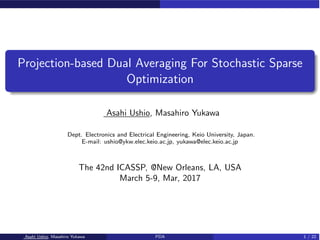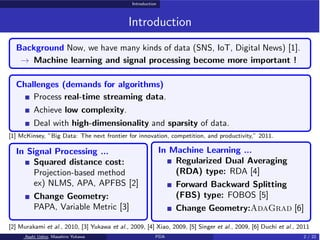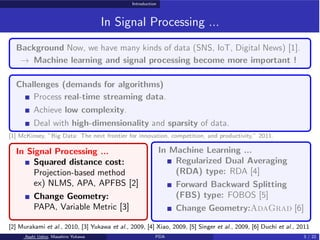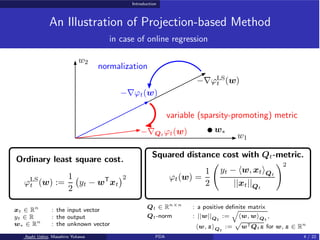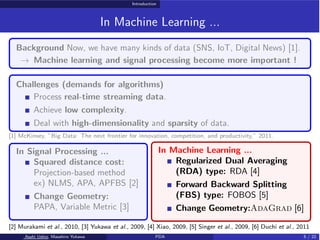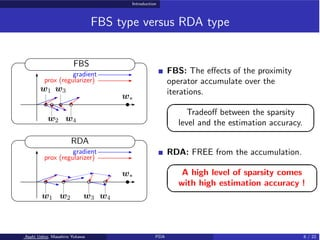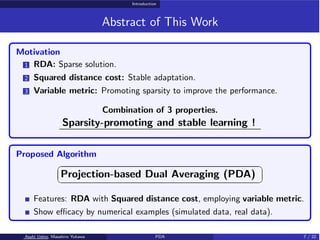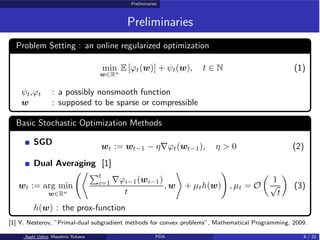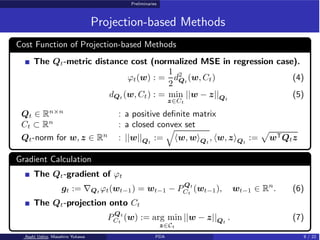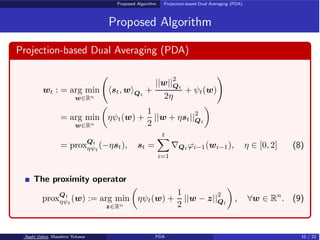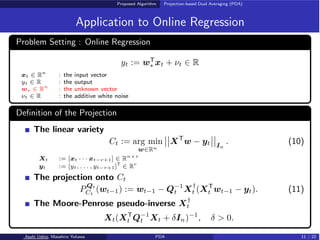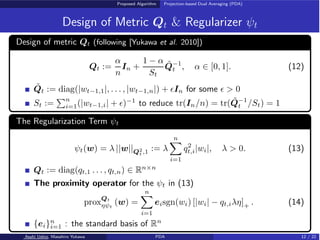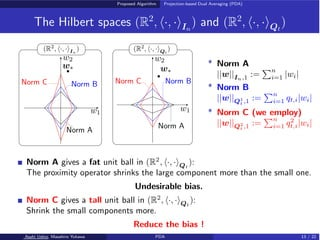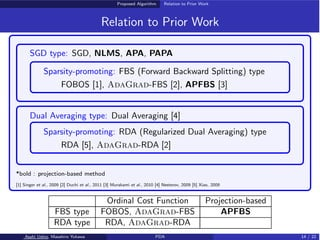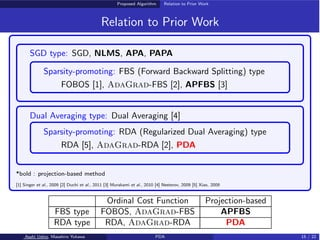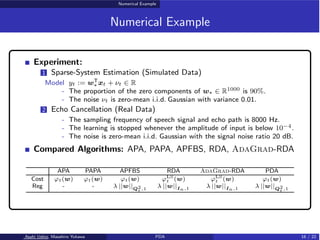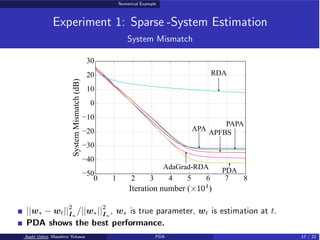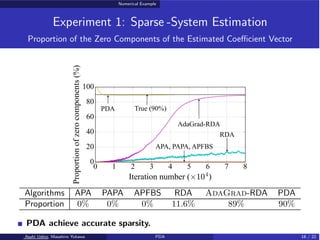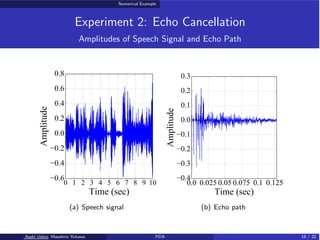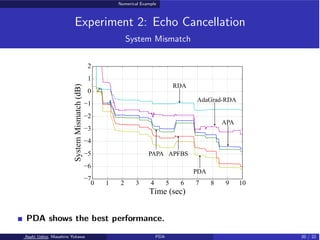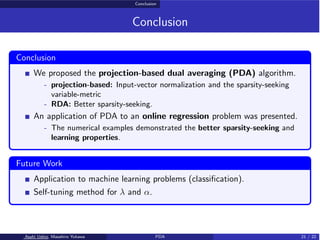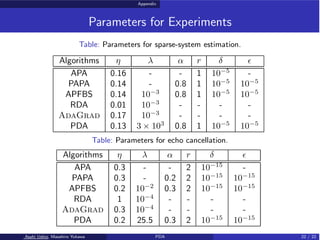1 of 22

### 2017-03, ICASSP, Projection-based Dual Averaging for Stochastic Sparse Optimization

• 1. Projection-based Dual Averaging For Stochastic Sparse Optimization Asahi Ushio, Masahiro Yukawa Dept. Electronics and Electrical Engineering, Keio University, Japan. E-mail: ushio@ykw.elec.keio.ac.jp, yukawa@elec.keio.ac.jp The 42nd ICASSP, @New Orleans, LA, USA March 5-9, Mar, 2017 Asahi Ushio, Masahiro Yukawa PDA 1 / 22
• 2. Introduction Introduction Background Now, we have many kinds of data (SNS, IoT, Digital News) . → Machine learning and signal processing become more important ! Challenges (demands for algorithms) Process real-time streaming data. Achieve low complexity. Deal with high-dimensionality and sparsity of data.  McKinsey, ”Big Data: The next frontier for innovation, competition, and productivity,” 2011. In Signal Processing ... Squared distance cost: Projection-based method ex) NLMS, APA, APFBS  Change Geometry: PAPA, Variable Metric  In Machine Learning ... Regularized Dual Averaging (RDA) type: RDA  Forward Backward Splitting (FBS) type: FOBOS  Change Geometry:AdaGrad   Murakami et al., 2010,  Yukawa et al., 2009,  Xiao, 2009,  Singer et al., 2009,  Duchi et al., 2011 Asahi Ushio, Masahiro Yukawa PDA 2 / 22
• 3. Introduction In Signal Processing ... Background Now, we have many kinds of data (SNS, IoT, Digital News) . → Machine learning and signal processing become more important ! Challenges (demands for algorithms) Process real-time streaming data. Achieve low complexity. Deal with high-dimensionality and sparsity of data.  McKinsey, ”Big Data: The next frontier for innovation, competition, and productivity,” 2011. In Signal Processing ... Squared distance cost: Projection-based method ex) NLMS, APA, APFBS  Change Geometry: PAPA, Variable Metric  In Machine Learning ... Regularized Dual Averaging (RDA) type: RDA  Forward Backward Splitting (FBS) type: FOBOS  Change Geometry:AdaGrad   Murakami et al., 2010,  Yukawa et al., 2009,  Xiao, 2009,  Singer et al., 2009,  Duchi et al., 2011 Asahi Ushio, Masahiro Yukawa PDA 3 / 22
• 4. Introduction An Illustration of Projection-based Method in case of online regression −∇ϕLS t (w) −∇ϕt(w) −∇Qt ϕt(w) w∗ normalization variable (sparsity-promoting) metric w2 w1 Ordinary least square cost. ϕLS t (w) := 1 2 ! yt − wT xt "2 Squared distance cost with Qt-metric. ϕt(w) = 1 2 # yt − ⟨w, xt⟩Qt ||xt||Qt \$2 xt ∈ Rn : the input vector yt ∈ R : the output w∗ ∈ Rn : the unknown vector Qt ∈ Rn×n : a positive definite matrix Qt-norm : ||w||Qt := ! ⟨w, w⟩Qt , ⟨w, z⟩Qt := " wTQtz for w, z ∈ Rn Asahi Ushio, Masahiro Yukawa PDA 4 / 22
• 5. Introduction In Machine Learning ... Background Now, we have many kinds of data (SNS, IoT, Digital News) . → Machine learning and signal processing become more important ! Challenges (demands for algorithms) Process real-time streaming data. Achieve low complexity. Deal with high-dimensionality and sparsity of data.  McKinsey, ”Big Data: The next frontier for innovation, competition, and productivity,” 2011. In Signal Processing ... Squared distance cost: Projection-based method ex) NLMS, APA, APFBS  Change Geometry: PAPA, Variable Metric  In Machine Learning ... Regularized Dual Averaging (RDA) type: RDA  Forward Backward Splitting (FBS) type: FOBOS  Change Geometry:AdaGrad   Murakami et al., 2010,  Yukawa et al., 2009,  Xiao, 2009,  Singer et al., 2009,  Duchi et al., 2011 Asahi Ushio, Masahiro Yukawa PDA 5 / 22
• 6. Introduction FBS type versus RDA type grad grad FBS RDA gradient gradient prox (regularizer) prox (regularizer) w∗ w∗ w1 w1 w2 w2 w3 w3 w4 w4 FBS: The eﬀects of the proximity operator accumulate over the iterations. ✎ ✍ ☞ ✌ Tradeoﬀ between the sparsity level and the estimation accuracy. RDA: FREE from the accumulation. ✎ ✍ ☞ ✌ A high level of sparsity comes with high estimation accuracy ! Asahi Ushio, Masahiro Yukawa PDA 6 / 22
• 7. Introduction Abstract of This Work Motivation 1 RDA: Sparse solution. 2 Squared distance cost: Stable adaptation. 3 Variable metric: Promoting sparsity to improve the performance. Combination of 3 properties. Sparsity-promoting and stable learning ! Proposed Algorithm ✞ ✝ ☎ ✆ Projection-based Dual Averaging (PDA) Features: RDA with Squared distance cost, employing variable metric. Show eﬃcacy by numerical examples (simulated data, real data). Asahi Ushio, Masahiro Yukawa PDA 7 / 22
• 8. Preliminaries Preliminaries Problem Setting : an online regularized optimization min w∈Rn E [ϕt(w)] + ψt(w), t ∈ N (1) ψt,ϕt : a possibly nonsmooth function w : supposed to be sparse or compressible Basic Stochastic Optimization Methods SGD wt := wt−1 − η∇ϕt(wt−1), η > 0 (2) Dual Averaging  wt := arg min w∈Rn #%&t i=1 ∇ϕi−1(wi−1) t , w ' + µth(w) \$ , µt = O ( 1 √ t ) (3) h(w) : the prox-function  Y. Nesterov, ”Primal-dual subgradient methods for convex problems”, Mathematical Programming, 2009. Asahi Ushio, Masahiro Yukawa PDA 8 / 22
• 9. Preliminaries Projection-based Methods Cost Function of Projection-based Methods The Qt-metric distance cost (normalized MSE in regression case). ϕt(w) : = 1 2 d2 Qt (w, Ct) (4) dQt (w, Ct) : = min z∈Ct ||w − z||Qt (5) Qt ∈ Rn×n : a positive definite matrix Ct ⊂ Rn : a closed convex set Qt-norm for w, z ∈ Rn : ||w||Qt := * ⟨w, w⟩Qt , ⟨w, z⟩Qt := + wTQtz Gradient Calculation The Qt-gradient of ϕt gt := ∇Qt ϕt(wt−1) = wt−1 − PQt Ct (wt−1), wt−1 ∈ Rn . (6) The Qt-projection onto Ct PQt Ct (w) := arg min z∈Ct ||w − z||Qt . (7) Asahi Ushio, Masahiro Yukawa PDA 9 / 22
• 10. Proposed Algorithm Projection-based Dual Averaging (PDA) Proposed Algorithm Projection-based Dual Averaging (PDA) wt : = arg min w∈Rn # ⟨st, w⟩Qt + ||w|| 2 Qt 2η + ψt(w) \$ = arg min w∈Rn ( ηψt(w) + 1 2 ||w + ηst|| 2 Qt ) = proxQt ηψt (−ηst), st = t , i=1 ∇Qt ϕi−1(wi−1), η ∈ [0, 2] (8) The proximity operator proxQt ηψt (w) := arg min z∈Rn ( ηψt(w) + 1 2 ||w − z|| 2 Qt ) , ∀w ∈ Rn . (9) Asahi Ushio, Masahiro Yukawa PDA 10 / 22
• 11. Proposed Algorithm Projection-based Dual Averaging (PDA) Application to Online Regression Problem Setting : Online Regression yt := wT ∗ xt + νt ∈ R xt ∈ Rn : the input vector yt ∈ R : the output w∗ ∈ Rn : the unknown vector νt ∈ R : the additive white noise Definition of the Projection The linear variety Ct := arg min w∈Rn - - - -XT w − yt - - - - In . (10) Xt := [xt · · · xt−r+1] ∈ Rn×r yt := [yt, . . . , yt−r+1]T ∈ Rr The projection onto Ct PQt Ct (wt−1) := wt−1 − Q−1 t X† t (XT t wt−1 − yt). (11) The Moore-Penrose pseudo-inverse X† t Xt(XT t Q−1 t Xt + δIn)−1 , δ > 0. Asahi Ushio, Masahiro Yukawa PDA 11 / 22
• 12. Proposed Algorithm Projection-based Dual Averaging (PDA) Design of Metric Qt & Regularizer ψt Design of metric Qt (following [Yukawa et al. 2010]) Qt := α n In + 1 − α St Q̃−1 t , α ∈ [0, 1]. (12) Q̃t := diag(|wt−1,1|, . . . , |wt−1,n|) + ϵIn for some ϵ > 0 St := &n i=1(|wt−1,i| + ϵ)−1 to reduce tr(In/n) = tr(Q̃−1 t /St) = 1 The Regularization Term ψt ψt(w) = λ ||w||Q2 t ,1 := λ n , i=1 q2 t,i|wi|, λ > 0. (13) Qt := diag(qt,1 . . . , qt,n) ∈ Rn×n The proximity operator for the ψt in (13) proxQt ηψt (w) = n , i=1 eisgn(wi) [|wi| − qt,iλη]+ . (14) {ei}n i=1 : the standard basis of Rn Asahi Ushio, Masahiro Yukawa PDA 12 / 22
• 13. Proposed Algorithm Projection-based Dual Averaging (PDA) The Hilbert spaces (R2 , ⟨·, ·⟩In ) and (R2 , ⟨·, ·⟩Qt ) (R2 , ⟨·, ·⟩Qt ) (R2 , ⟨·, ·⟩In ) Norm A Norm A Norm B Norm B Norm C Norm C w1 w2 w2 w1 w∗ w∗ * Norm A ||w||In,1 := &n i=1 |wi| * Norm B ||w||Q1 t ,1 := &n i=1 qt,i|wi| * Norm C (we employ) ||w||Q2 t ,1 := &n i=1 q2 t,i|wi| Norm A gives a fat unit ball in (R2 , ⟨·, ·⟩Qt ): The proximity operator shrinks the large component more than the small one. Undesirable bias. Norm C gives a tall unit ball in (R2 , ⟨·, ·⟩Qt ): Shrink the small components more. Reduce the bias ! Asahi Ushio, Masahiro Yukawa PDA 13 / 22
• 14. Proposed Algorithm Relation to Prior Work Relation to Prior Work SGD type: SGD, NLMS, APA, PAPA Sparsity-promoting: FBS (Forward Backward Splitting) type FOBOS , AdaGrad-FBS , APFBS  Dual Averaging type: Dual Averaging  Sparsity-promoting: RDA (Regularized Dual Averaging) type RDA , AdaGrad-RDA  *bold : projection-based method  Singer et al., 2009  Duchi et al., 2011  Murakami et al., 2010  Nesterov, 2009  Xiao, 2009 Ordinal Cost Function Projection-based FBS type FOBOS, AdaGrad-FBS APFBS RDA type RDA, AdaGrad-RDA Asahi Ushio, Masahiro Yukawa PDA 14 / 22
• 15. Proposed Algorithm Relation to Prior Work Relation to Prior Work SGD type: SGD, NLMS, APA, PAPA Sparsity-promoting: FBS (Forward Backward Splitting) type FOBOS , AdaGrad-FBS , APFBS  Dual Averaging type: Dual Averaging  Sparsity-promoting: RDA (Regularized Dual Averaging) type RDA , AdaGrad-RDA , PDA *bold : projection-based method  Singer et al., 2009  Duchi et al., 2011  Murakami et al., 2010  Nesterov, 2009  Xiao, 2009 Ordinal Cost Function Projection-based FBS type FOBOS, AdaGrad-FBS APFBS RDA type RDA, AdaGrad-RDA PDA Asahi Ushio, Masahiro Yukawa PDA 15 / 22
• 16. Numerical Example Numerical Example Experiment: 1 Sparse-System Estimation (Simulated Data) Model yt := wT ∗ xt + νt ∈ R - The proportion of the zero components of w∗ ∈ R1000 is 90%. - The noise νt is zero-mean i.i.d. Gaussian with variance 0.01. 2 Echo Cancellation (Real Data) - The sampling frequency of speech signal and echo path is 8000 Hz. - The learning is stopped whenever the amplitude of input is below 10−4. - The noise is zero-mean i.i.d. Gaussian with the signal noise ratio 20 dB. Compared Algorithms: APA, PAPA, APFBS, RDA, AdaGrad-RDA APA PAPA APFBS RDA AdaGrad-RDA PDA Cost ϕt(w) ϕt(w) ϕt(w) ϕLS t (w) ϕLS t (w) ϕt(w) Reg - - λ ||w||Q2 t ,1 λ ||w||In,1 λ ||w||In,1 λ ||w||Q2 t ,1 Asahi Ushio, Masahiro Yukawa PDA 16 / 22
• 17. Numerical Example Experiment 1: Sparse -System Estimation System Mismatch APA PAPA APFBS RDA AdaGrad-RDA PDA ||w∗ − wt|| 2 In /||w∗|| 2 In , w∗ is true parameter, wt is estimation at t. PDA shows the best performance. Asahi Ushio, Masahiro Yukawa PDA 17 / 22
• 18. Numerical Example Experiment 1: Sparse -System Estimation Proportion of the Zero Components of the Estimated Coeﬃcient Vector RDA AdaGrad-RDA PDA True (90%) APA, PAPA, APFBS Algorithms APA PAPA APFBS RDA AdaGrad-RDA PDA Proportion 0% 0% 0% 11.6% 89% 90% PDA achieve accurate sparsity. Asahi Ushio, Masahiro Yukawa PDA 18 / 22
• 19. Numerical Example Experiment 2: Echo Cancellation Amplitudes of Speech Signal and Echo Path (a) Speech signal (b) Echo path Asahi Ushio, Masahiro Yukawa PDA 19 / 22
• 20. Numerical Example Experiment 2: Echo Cancellation System Mismatch APA PAPA APFBS RDA AdaGrad-RDA PDA PDA shows the best performance. Asahi Ushio, Masahiro Yukawa PDA 20 / 22
• 21. Conclusion Conclusion Conclusion We proposed the projection-based dual averaging (PDA) algorithm. - projection-based: Input-vector normalization and the sparsity-seeking variable-metric - RDA: Better sparsity-seeking. An application of PDA to an online regression problem was presented. - The numerical examples demonstrated the better sparsity-seeking and learning properties. Future Work Application to machine learning problems (classification). Self-tuning method for λ and α. Asahi Ushio, Masahiro Yukawa PDA 21 / 22
• 22. Appendix Parameters for Experiments Table: Parameters for sparse-system estimation. Algorithms η λ α r δ ϵ APA 0.16 - - 1 10−5 - PAPA 0.14 - 0.8 1 10−5 10−5 APFBS 0.14 10−3 0.8 1 10−5 10−5 RDA 0.01 10−3 - - - - AdaGrad 0.17 10−3 - - - - PDA 0.13 3 × 103 0.8 1 10−5 10−5 Table: Parameters for echo cancellation. Algorithms η λ α r δ ϵ APA 0.3 - - 2 10−15 - PAPA 0.3 - 0.2 2 10−15 10−15 APFBS 0.2 10−2 0.3 2 10−15 10−15 RDA 1 10−4 - - - - AdaGrad 0.3 10−4 - - - - PDA 0.2 25.5 0.3 2 10−15 10−15 Asahi Ushio, Masahiro Yukawa PDA 22 / 22